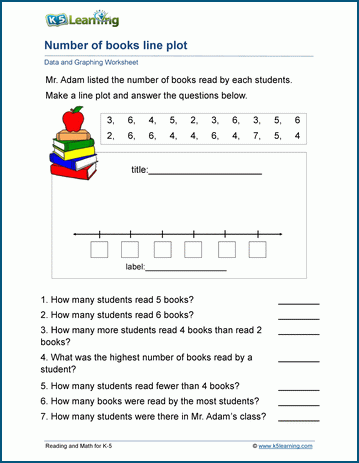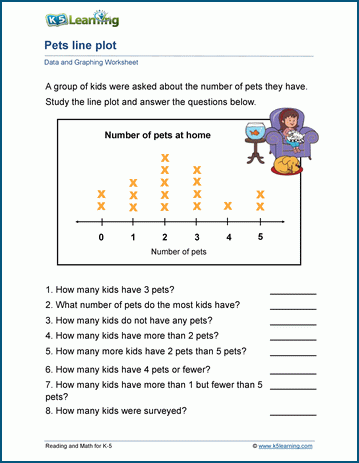# How to Make and Interpret Line Plots

## Making line plots

Here’s one approach to teaching your kids to make line plots.

Choose a data set

Select a simple set of data that is easy for third graders to understand. For example, you might survey students on their favorite colors or the number of pets they have.

Collect data

Have students collect data from their classmates or from a predetermined set.

Organize the data

List the categories along the bottom (X-axis) and determine the range of the data.

Create a number line

Draw a horizontal line (the number line) above the categories. Mark the line with the range of the data.

Plot the data

For each piece of data, make an "X" above the appropriate category on the number line.

Label the axes

Label the X-axis with the categories, and the Y-axis (if necessary) with the frequency or count.

Title the line plot

Give the line plot a title that reflects the data being shown.

## Interpreting line plots

Here’s one approach to teaching your kids to interpret line plots.

Counting

Teach students to count the Xs above each category to determine the frequency or count.

Understanding the data

Discuss what the line plot reveals about the data. For example, which category has the most/least occurrences?

Analyzing trends

Encourage students to identify any patterns or trends in the data. Are there any categories that are more popular than others?

Ask questions related to the line plot. For example, "How many students chose red as their favorite color?" or "What is the most common number of pets among students?"

Using the data

Discuss real-world scenarios where understanding data is important. For example, if the data is about favorite fruits, you might discuss planning a class fruit party based on preferences.

## Grade 2 line plots worksheets

In our grade 2 data and graphing section, we have a page for kids to work on reading line plots and making line plots.## Grade 3 line plots worksheets

These worksheets provide students with data to enter into line plots.We also have worksheets for grade 3 students to read and interpret line plots, based on whole numbers and fractional bases.## Some tips on working with line plots

Here are some more tips when you work with your kids with line plots.

Visuals

Use visuals and color to make the line plot more engaging.

Hands-On Activities

Consider hands-on activities, such as having students physically place objects on a line plot.

Relevance

Relate the line plot to the students' lives to make it more relevant and interesting.

Review

Regularly review the concepts of line plots and interpretation to reinforce learning.

Remember to adapt the complexity of the line plots and questions based on the students' understanding and comfort level with the concepts.Become a Member

This content is available to members only.